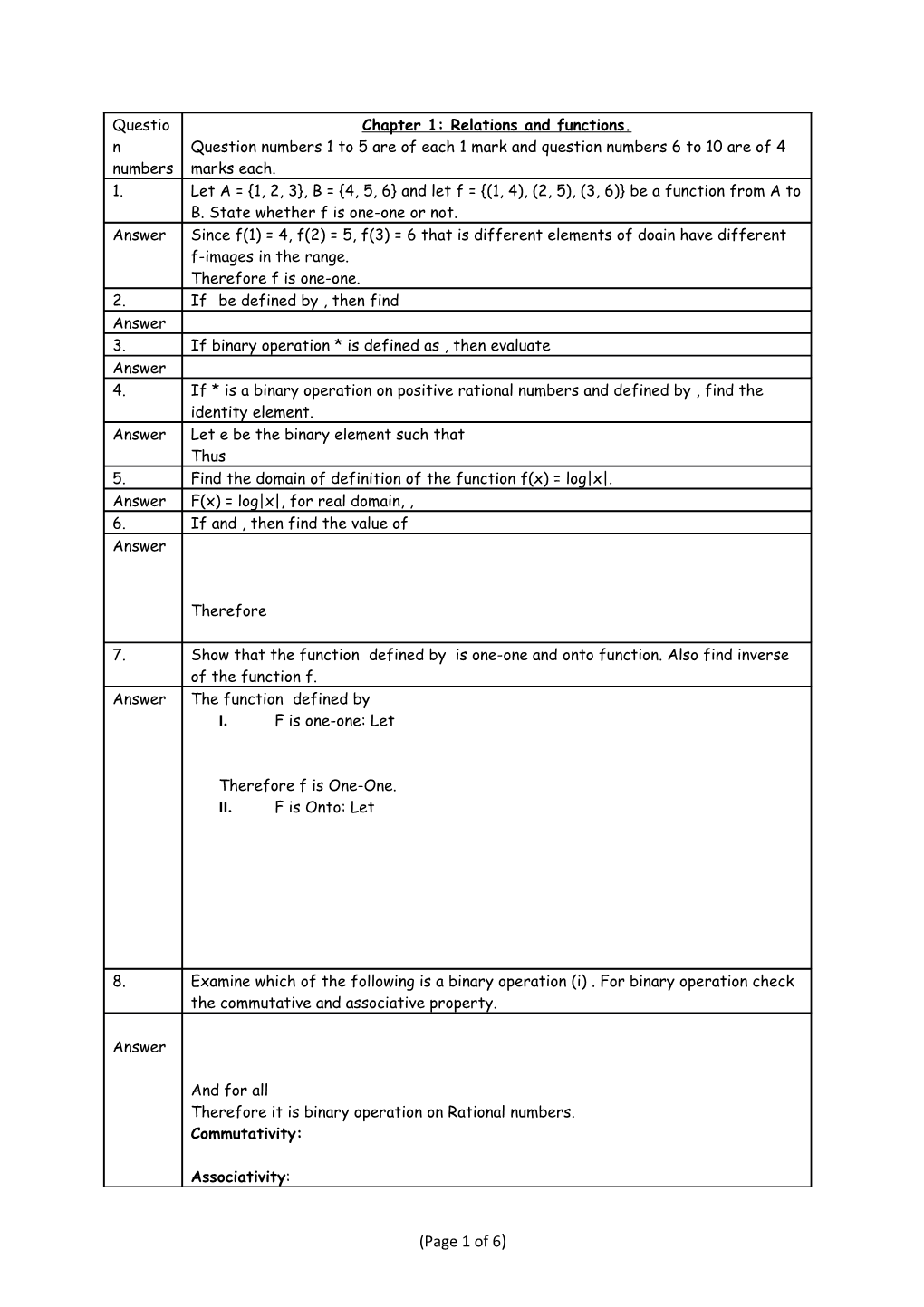# F Is One-One: LetQuestion numbers / Chapter 1: Relations and functions.
Question numbers 1 to 5 are of each 1 mark and question numbers 6 to 10 are of 4 marks each.
Let A = {1, 2, 3}, B = {4, 5, 6} and let f = {(1, 4), (2, 5), (3, 6)} be a function from A to B. State whether f is one-one or not.
Answer / Since f(1) = 4, f(2) = 5, f(3) = 6 that is different elements of doain have different f-images in the range.
Therefore f is one-one.
If be defined by , then find
If binary operation * is defined as , then evaluate
If * is a binary operation on positive rational numbers and defined by , find the identity element.
Answer / Let e be the binary element such that
Thus
Find the domain of definition of the function f(x) = log|x|.
Answer / F(x) = log|x|, for real domain, ,
If and , then find the value of

Therefore

Show that the function defined by is one-one and onto function. Also find inverse of the function f.
Answer / The function defined by
1. F is one-one: Let

Therefore f is One-One.
1. F is Onto: Let

Examine which of the following is a binary operation (i) . For binary operation check the commutative and associative property.

And for all
Therefore it is binary operation on Rational numbers.
Commutativity:

Associativity:

Therefore,
Or binary operation is not Associative.
Let X be non-empty set. P(X) be its power set. Let ‘*’ be an operation defined on elements of P(x) by , then (i) Prove that * is a binary operation in P(X). (ii) Is * commutative? (iii) Is * Associative? (iv) Find the identity element in P(X) w.r.t *.
(i)Let A and B are any two non-empty subsets of X, then we know that
Now for any two non empty elements of P(X), we have
Therefore, ‘*’ is binary operation in P(X).
(ii)For any two non-empty subsets A and B of X, then we know that

Therefore ‘*’ is commutative.
(iii)For any three non-empty subsets A, B, C
We have
Therefore * is associative.
(iv)Let I be the identity element in P(X).
A*I = I*A = A

Therefore

Thus I is the identity element.
Given a non-empty set X, let be define as . Show that the empty set is the identity fr the operation * and all the elements A of P(X) are invertible with
Answer / Given let be define as
I Part: Let

II Part: Let

Chapter 2: Inverse Trigonometric Functions
Question numbers 11 to 15 are of each 1 mark and question numbers 16 to 20 are of 4 marks each.
What is the principle value of ?

Find the value of

If , then find the principle value of .

Express

For what value of x,

Find the value of

Solve for x:

Prove that

If

If

Square both the sides

Chapter 3: Matrices
Question numbers 21 to 25 are of each 1 mark and question numbers 26 to 30 are of 4 marks each and question numbers 31 to 35 are of 6 marks each.
If

Construct a 2 x 2 matrix whose element
If
If
If

If

Hence proved.
Pre-multiplying

Represent the given matrix as the sum of symmetric and skew symmetric matrix

Let

Let

Hence the result.
If , where n is any positive integer.
Answer / We shall prove it by using principle of Mathematical Induction.
For n = 1, , which is true.
Therefore given statement is true for n = 1.
Let us suppose that given statement is true for n = k.

Let us take n = k + 1

which is true for n = k +1
Therefore by PMI, it is true for every positive integer k.
Find the values of x, y and z if the given matrix A satisfy the equation A.A’ =I where
Given that AA’=I

Prove that inverse of every square matrix, if it exists, is unique.
Answer / Let ‘A’ be any invertible square matrix of order n.
Let B and C are two inverse of A.
Therefore; AB = BA = I - - - - - (1)
And AC = CA = I ------(2)
From (1), since AB = I
Pre-multiply it by C, we get,
C(AB) = CI
Or (CA)B = C [Since C(AB) = (CA)B and CI = C]
Or IB = C [from (2), CA = I]
Or B = C
Hence inverse of a matrix is unique.
Using elementary operations find the inverse of
Answer / Using row operations; therefore, A = IA

Therefore
Using elementary operations find the inverse of
Answer / Let us apply row operations, that is A = IA
Therefore

Therefore
Using elementary operations find the inverse of